Courses

# Combinational Logic Circuits - 2

## 20 Questions MCQ Test Topicwise Question Bank for Electrical Engineering | Combinational Logic Circuits - 2

Description
This mock test of Combinational Logic Circuits - 2 for Electrical Engineering (EE) helps you for every Electrical Engineering (EE) entrance exam. This contains 20 Multiple Choice Questions for Electrical Engineering (EE) Combinational Logic Circuits - 2 (mcq) to study with solutions a complete question bank. The solved questions answers in this Combinational Logic Circuits - 2 quiz give you a good mix of easy questions and tough questions. Electrical Engineering (EE) students definitely take this Combinational Logic Circuits - 2 exercise for a better result in the exam. You can find other Combinational Logic Circuits - 2 extra questions, long questions & short questions for Electrical Engineering (EE) on EduRev as well by searching above.
QUESTION: 1

Solution:
QUESTION: 2

Solution:
QUESTION: 3

### The combinational circuit shown below has three data lines D1, D2,and D3 while one select line S.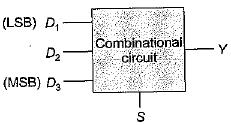When the control line is high, the circuit is to detect when one of the data lines has ‘1’ on it. No more than one data line will ever have '1' on it. When the control line is low, the circuit will output ‘O’, regardless of what is on the data lines. The output Y of the combinational circuit will be:

Solution:

The truth table for the given problem is shown below: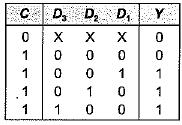The K-map for the above truth table is shown below: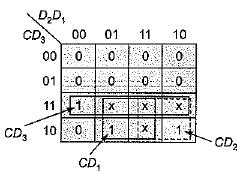Thus output, Y = CD3 + CD2 + CD1
= C(D1 + D+ D3)

QUESTION: 4

Figure below shows an automobile alarm circuit used to detect certain undesirable conditions. The three switches are used to indicate the status of the door by the driver’s seat, the ignition and the head lights, respectively.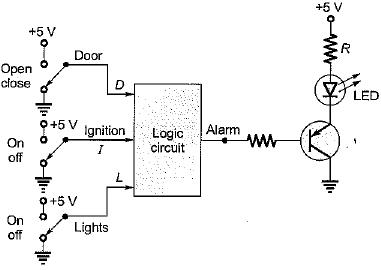What is the output of the above logic circuit with these three switches (D, I and L) as inputs so that the alarm will be activated whenever either of the following condition exists:
The headlights are ON while the ignition is OFF.
The door is open while the ignition is ON.

Solution:

Let us consider D for door, I for ignition and L for light. Then, conditions to activate the alarm are:
(i) The headlights are ON while the ignition is OFF
i.e. L = 1, I = 0 and D may be anything.
(ii) The door is open while the ignition is ON. i.e. D = 1 , I = 1, L may be anything.
Also, alarm will sound if logic circuit output is zero.
Therefore, output (V) for above condition is zero and for rest of the condition it is 1 which is shown in following truth table.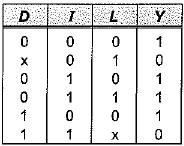K-map for above truth table is shown below.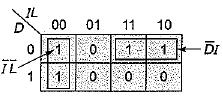Thus, the output of the given logic circuit is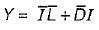QUESTION: 5

A logic circuit takes 4-BCD inputs {A, B, C and D) to give an output F. Output F is '1' if the input is an invalid BCD-code. The number of two input NAND gates required to implement the output Y is

Solution:

Invalid BCD inputs are from decimal 10 to 11. The K-map is shown below: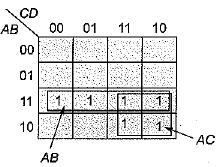Thus, output F = AB + AC, which can be implemented using three 2-input NAND gates as shown below,.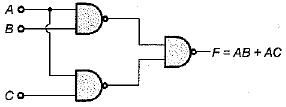QUESTION: 6

A 1-bit full adder takes 15 ns to generate carry out bit while 35 ns for the sum bit. If we design a 4-bit adder using cascade connection of four 1-bit full-adder, then the maximum number of additions that can be performed by this 4-bit adder will be:

Solution:

Let the binary inputs to be added be (A4 A3 A2 A1) and (B4 B3 B2 B1). A 4-bit adder using cascade connection of four 1-bit full adder is shown below. Here, C represents for carry and Sfor sum.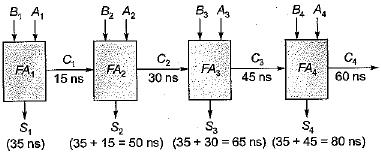It is clear from above circuit that each addition requires 80 ns.
Therefore, maximum number of additions that can be performed by this 4-bit adder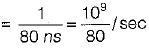= 125 x 105 additions/sec

QUESTION: 7

The number of two-input multiplexers required to implement an EX-NOR and a NAND gate are respectively

Solution:

Let the two inputs variations be Xand Y.
EX-NOR gate using 2 x 1 MUX: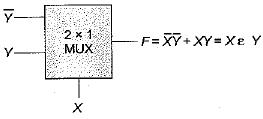Here, we require two 2 x 1 MUX.
NAND g a te using 2 x 1 MUX: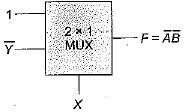Thus, we require two number of 2 x 1 MUX for NAND gate implementation.

QUESTION: 8

The circuit shown below represents a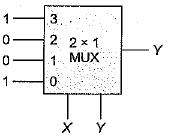Solution:

Output,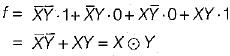= EX-NOR gate

QUESTION: 9

The logic expression f(A, B, C) = πM (0, 3,5) is required to be implemented using a 4 x 1 MUX as shown below.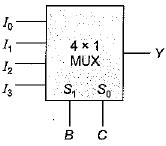The combinations of the input to this 4 x 1 MUX circuit would be

Solution:

Given, logic expression is
f(A, B, C) = πM(0,3,5) = ∑m(1,2, 4, 6,7).
Here, B and C has to be control inputs while A as data inputs. We form the table as shown below: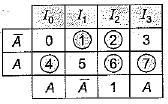Thus,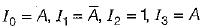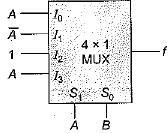QUESTION: 10

A full adder circuit can be implemented using:
1. One 3 x 8 decoder and two OR gates.
2. One 3 x 8 decoder, one OR gate and a NOT gate.
3. Two half adders and one OR gate.
4. Two half adders and one NOT gate.
5. Nine NAND/NOR gates.
6. 6 NAND/NOR gates.
Select the correct code from the given options.

Solution:

A full adder is used to add three input bits (A, R and C) to give two outputs namely sum and carry as shown below: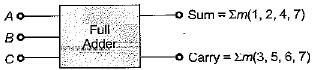For its implementation we require either of the following:

• One 3 x 8 decoder and two OR gates.
• Two half adders and one OR gate.
• Nine NAND/NOR gates.
QUESTION: 11

To add two 4-bit numbers using parallel adder circuit we require

Solution:

To add two n-bit numbers using parallel adder circuit, we require
(n - 1) full adders and 1-half adder
or
n-full adders
or
(2n -1) half adders and (n - 1) OR gates Thus, to add two 4-bit numbers using parallel adder circuit, we require
3- full adder and 1 -half adder
or
4- full adders
or
7-half adder and 3 OR gates
Hence, option (d) is correct.

QUESTION: 12

A combinational circuit has three inputs namely A, B and C (A being MSB and C being LSB).
Match List-I (Combinational Circuit outputs) with List-II (Logic expressions) and select the correct answer using the codes given below the lists:
List-I
A. Full adder carry output
B. Full subtractor borrow output
C. Sum output of full adder or difference output of full subtractor
List-II
1.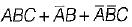2.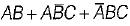3.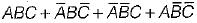Codes:
A B C
(a) 2 1 3
(b) 1 2 3
(c) 1 3 2
(d) 2 3 1

Solution: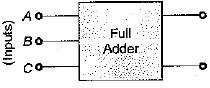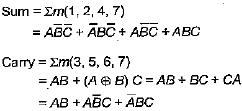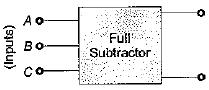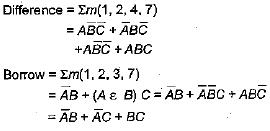QUESTION: 13

The correction to be appiied in decimal adder to the generated sum is

Solution:

The correction to be applied in decimal adder to the generated sum is 00110. When the four bit sum is more than 9, then the sum is invalid, in such cases, +6 (i.e. 0110) is added to the four bit sum to skip the six invalid states, if a carry is generated when adding 6, the carry is added to the next four bit group.

QUESTION: 14

The gates required to build a half adder are:

Solution:

The gates required to build a half adder are EX-OR gate and AND gate. Figure below shows the logic diagram of half adder.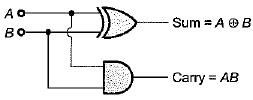QUESTION: 15

The code where all successive numbers differ from their preceding number by single bit is

Solution:

The code where all successive numbers differ from their preceding number by single bit is Gray code. (It is an unweighted code. The most important characteristic of this code is that only a single bit change occurs when going from one code number to next).

QUESTION: 16

A device which changes from serial data to parallel data is

Solution:

The device which changes from serial data to parallel data is demultiplexer because it takes in data from one line and directs it to any of its A/outputs depending on the status of the select inputs.

QUESTION: 17

A device which converts BCD to Seven Segment is called

Solution:

A decoder converts binary words into alphanumeric characters i.e. it converts BCD to seven segment.

QUESTION: 18

The logic circuit shown below converts a binary code x1 xx3 into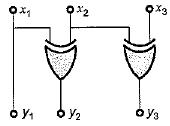Solution:

From given circuit, we have:
y1 = x1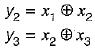Thus, the given circuit will convert binary code x1, x2, xinto Gray code y1 y2 y3.

QUESTION: 19

The logic circuit shown below can be minimized to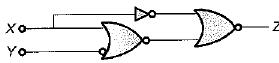Solution:

From given circuit, output is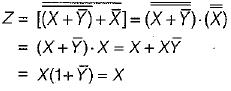QUESTION: 20

When the set of input data to an even parity generator is 0111, the output will be

Solution:

In even parity generator if number of ‘1’ is odd then output wifi be zero. Here, input 0111 has odd number of 1's, therefore output of even parity generator will be zero.